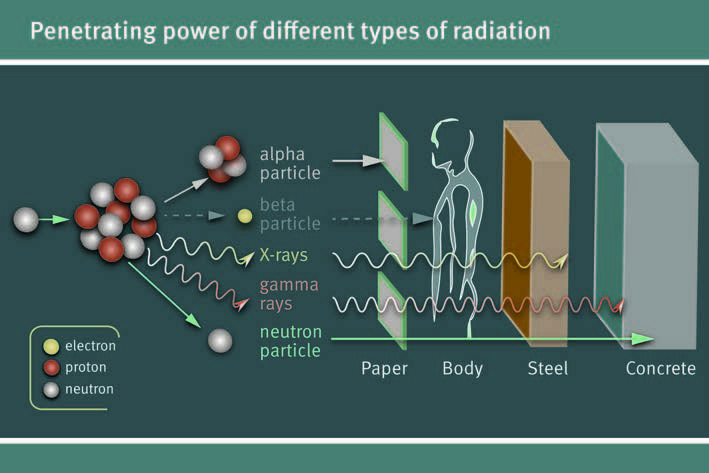# Dosage

Radiation dose is the amount of energy absorbed from being exposed to different forms of ionizing radiation. Radiation dose has three quantity definitions: absorbed, equivalent and effective dose.

• Absorbed dose is defined as the total energy absorbed by a material per unit mass. The non-SI unit Rad was first used to measure absorbed dose. Today absorbed dose is most commonly measured in gray or Gy. Gy can be used to measure any form of radiation except for the biological effects of the different forms of radiations.
• Equivalent dose is used to measure the biological effects of radiation on living matter. The different types of radiation such as alpha, beta and gamma determine the severity of the biological effect. Dose equivalent was once measured using the non-SI unit rem but is now widely measured using the SI unit sievert or Sv.
• Effective dose measures the amounts of damage different tissues have from radiation based on the dose equivalent. Like equivalent dose, effective dose is measured in Sv. Some tissues are more susceptible to radiation than others. Effective dose is used most commonly in medicine, as it is important for example to know how damaged one organ is compared to another.Figure 1: Penetrating power of different types of radiation. Penetrating power contributes to which organs are affected by different types of radiation.

Dosage doesn't tell the whole story, the effect of the dose depends strongly on what type of radiation is being absorbed such as alpha, beta and gamma as shown in Figure 1.

Dosage and the different quantity definitions do not represent the dose rate which is defined as the amount of accumulated radiation over time. For example living at sea level for a year is equivalent to 280 μSv and living at sea level for two years would be 560μSv. The interactive prezi below shows the amount of micro sieverts per year one can be exposed to based on their yearly activity. Note that while even food gives some radioactive dose the dosage that people receive is well below the level where harmful biological effects occur.

The difference between the different types of radiation is their energy levels, which in turn, have different effects on material. Alpha, beta and neutron radiation are considered to be in the form of particles while gamma rays and x-rays are in the form of waves. For example, alpha particles have the highest charge of all the radiation types and are therefore the most damaging due to their two charged protons and two neutrons. More info is available on the topic of charged particles and their effects on radiation levels and more on the different radiation doses. Calculate your annual radiation dose.

## Authors and Editors

Celeste Pomerantz, Jason Donev
Last updated: August 29, 2017
Get Citation